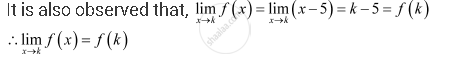Share

# Examine the Following Functions for Continuity. F (X) = X – 5 - CBSE (Commerce) Class 12 - Mathematics

#### Question

Examine the following functions for continuity.

f (x) = x – 5

#### Solution

The given function is f (x) = x – 5

It is evident that f is defined at every real number k and its value at k is k − 5.Hence, f is continuous at every real number and therefore, it is a continuous function.

Is there an error in this question or solution?

#### APPEARS IN

NCERT Solution for Mathematics Textbook for Class 12 (2018 to Current)
Chapter 5: Continuity and Differentiability
Q: 3.1 | Page no. 159

#### Video TutorialsVIEW ALL 

Solution Examine the Following Functions for Continuity. F (X) = X – 5 Concept: Continuous Function of Point.
S